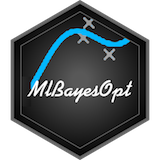# MlBayesOpt## Overview

This is an R package to tune hyperparameters for machine learning algorithms using Bayesian Optimization based on Gaussian Processes. Algorithms currently supported are: Support Vector Machines, Random Forest, and XGboost.

## Why MlBayesOpt ?

### Easy to write

It’s very easy to write Bayesian Optimaization function, but you also able to customise your model very easily. You have only to specify the data and the column name of the label to classify.

On XgBosst functions, your data frame is automatically transformed into `xgb.DMatrix` class.

### Any label class is OK

Any class (character, integer, factor) of label column is OK. The class of the label column is automatically transformed.

## Installation

You can install MlBayesOpt from github with:

``````# install.packages("githubinstall")
githubinstall::githubinstall("MlBayesOpt")

# install.packages("devtools")
devtools::install_github("ymattu/MlBayesOpt")``````

## Data

### Small Fashion MNIST

`fashion_train` and `fashion_test` are data reproduced from Fashion-MNIST. Each data has 1,000 rows and 784 feature column, and 1 label column named `y`.

`fashion` is a data made by the function `dplyr::bind_rows(fashion_train, fashion_test)`.

### iris

`iris_train` and `iris_test` are included in this pacakge. `iris_train` is odd-numbered rows of `iris` data, and `iris_test`is even-numbered rows of `iris` data.

## Example

### 3-fold cross validation for `iris` data, using SVM.

``````library(MlBayesOpt)

set.seed(71)
res0 <- svm_cv_opt(data = iris,
label = Species,
n_folds = 3,
init_points = 10,
n_iter = 1)
#> elapsed = 0.05   Round = 1   gamma_opt = 3.3299  cost_opt = 11.7670  Value = 0.9333
#> elapsed = 0.14   Round = 2   gamma_opt = 5.5515  cost_opt = 76.1740  Value = 0.9067
#> elapsed = 0.01   Round = 3   gamma_opt = 3.2744  cost_opt = 14.1882  Value = 0.9400
#> elapsed = 0.01   Round = 4   gamma_opt = 2.1175  cost_opt = 76.6932  Value = 0.9200
#> elapsed = 0.01   Round = 5   gamma_opt = 3.1619  cost_opt = 84.2154  Value = 0.9600
#> elapsed = 0.01   Round = 6   gamma_opt = 9.4727  cost_opt = 77.6772  Value = 0.8933
#> elapsed = 0.01   Round = 7   gamma_opt = 6.6175  cost_opt = 13.3914  Value = 0.9267
#> elapsed = 0.01   Round = 8   gamma_opt = 8.8943  cost_opt = 80.5955  Value = 0.8733
#> elapsed = 0.01   Round = 9   gamma_opt = 3.3808  cost_opt = 89.6793  Value = 0.9333
#> elapsed = 0.01   Round = 10  gamma_opt = 4.3481  cost_opt = 92.6987  Value = 0.9000
#> elapsed = 0.01   Round = 11  gamma_opt = 2.9508  cost_opt = 84.8600  Value = 0.9467
#>
#>  Best Parameters Found:
#> Round = 5    gamma_opt = 3.1619  cost_opt = 84.2154  Value = 0.9600``````

### 3-fold cross validation for `iris` data, using Xgboost.

``````res0 <- xgb_cv_opt(data = iris,
label = Species,
objectfun = "multi:softmax",
evalmetric = "mlogloss",
n_folds = 3,
classes = 3,
init_points = 10,
n_iter = 1)
#> elapsed = 0.07   Round = 1   eta_opt = 0.7235    max_depth_opt = 5.0000  nrounds_opt = 148.7789  subsample_opt = 0.9646  bytree_opt = 0.4860 Value = -0.6348
#> elapsed = 0.12   Round = 2   eta_opt = 0.5299    max_depth_opt = 6.0000  nrounds_opt = 100.5166  subsample_opt = 0.4912  bytree_opt = 0.5438 Value = -0.5967
#> elapsed = 0.01   Round = 3   eta_opt = 0.8751    max_depth_opt = 5.0000  nrounds_opt = 145.5496  subsample_opt = 0.7413  bytree_opt = 0.4354 Value = -0.6512
#> elapsed = 0.01   Round = 4   eta_opt = 0.4943    max_depth_opt = 5.0000  nrounds_opt = 101.2015  subsample_opt = 0.4600  bytree_opt = 0.7854 Value = -0.2085
#> elapsed = 0.01   Round = 5   eta_opt = 0.3203    max_depth_opt = 5.0000  nrounds_opt = 100.0397  subsample_opt = 0.3928  bytree_opt = 0.9258 Value = -0.1856
#> elapsed = 0.01   Round = 6   eta_opt = 0.1636    max_depth_opt = 5.0000  nrounds_opt = 112.8716  subsample_opt = 0.7814  bytree_opt = 0.8673 Value = -0.3862
#> elapsed = 0.01   Round = 7   eta_opt = 0.1895    max_depth_opt = 5.0000  nrounds_opt = 150.2979  subsample_opt = 0.2824  bytree_opt = 0.8784 Value = -0.3758
#> elapsed = 0.01   Round = 8   eta_opt = 0.3846    max_depth_opt = 5.0000  nrounds_opt = 147.7906  subsample_opt = 0.7400  bytree_opt = 0.6732 Value = -0.2351
#> elapsed = 0.01   Round = 9   eta_opt = 0.5668    max_depth_opt = 6.0000  nrounds_opt = 105.0991  subsample_opt = 0.2095  bytree_opt = 0.6461 Value = -0.2348
#> elapsed = 0.01   Round = 10  eta_opt = 0.6958    max_depth_opt = 4.0000  nrounds_opt = 139.9589  subsample_opt = 0.3209  bytree_opt = 0.8865 Value = -0.0585
#> elapsed = 0.01   Round = 11  eta_opt = 0.9098    max_depth_opt = 5.0000  nrounds_opt = 70.0000   subsample_opt = 0.8436  bytree_opt = 0.9599 Value = -0.0500
#>
#>  Best Parameters Found:
#> Round = 11   eta_opt = 0.9098    max_depth_opt = 5.0000  nrounds_opt = 70.0000   subsample_opt = 0.8436  bytree_opt = 0.9599 Value = -0.0500``````

## For Details

See the vignette (Coming Soon!)

## ToDo

• [x] Make functions to execute cross validation
• [ ] Fix minor bugs